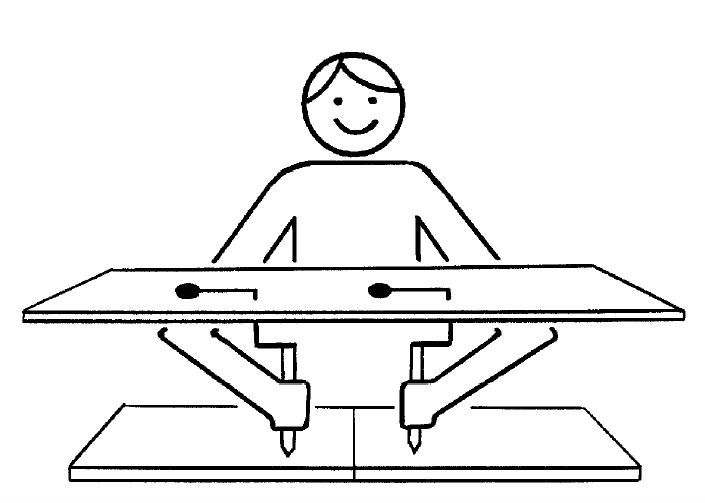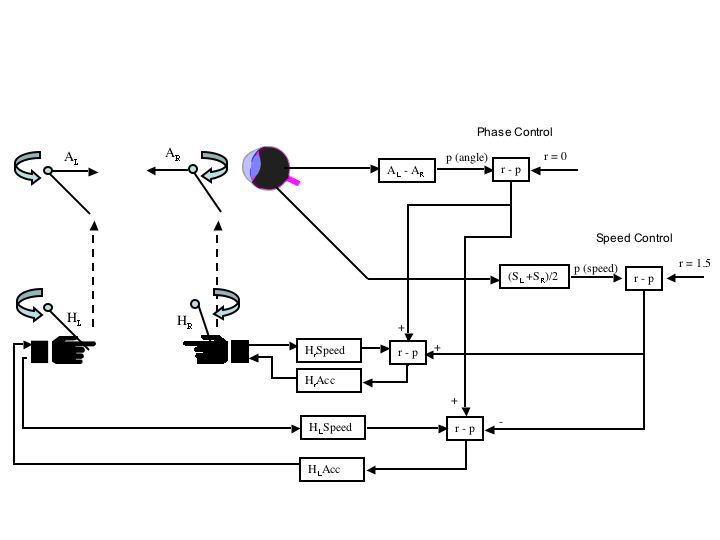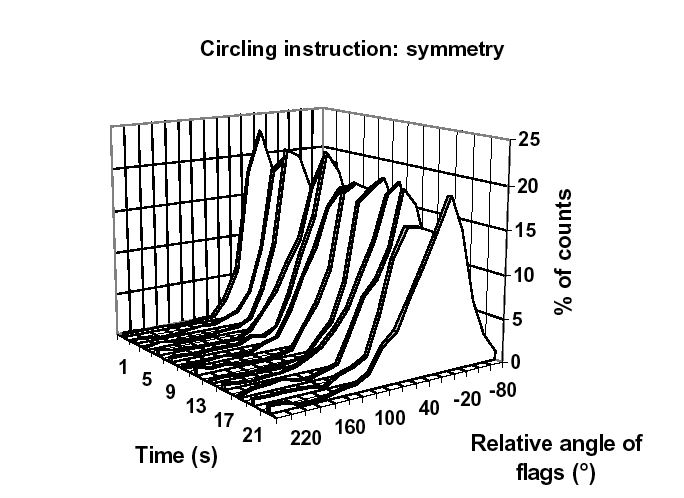### The PCT Model of Bimanual Coordination

This is a demonstration of a model of the behavior observed in a bimanual control experiment described in Mechsner, F., Kerzel, K., Knoblich, G. and Prinz, W. (2001) Perceptual basis of bimanual coordination, Nature, 414, 69-73 In that experiment participants were asked to keep two flag circling in symmetry by rotating corresponding left and right handles (see Figure 1 below). Subjects were able to produce this result despite the fact that right and left hand handle movements did not have equivalent effects on rotation of the corresponding flags. While there was a 1:1 relationship between left hand rotation and left flag rotation, there was only a .75:1 relationship between right hand rotation and right flag rotation. Therefore, in order to make the flags circle in symmetry the hands have to move at asymmetrical rates. Based on these results, Mechsner et al concluded that the motor outputs that move the handles are organized around the control of the perception of rotation of the flags, not control of the output movements that affect flag rotation. The model demonstrated in the program above is a mechanistic implementation of this idea.Figure 1

The model is shown in Figure 2 below. It's a two level perceptual control model. There are two high level systems, one controlling the relative angle between the left and right flags (Al-Ar) and the other controlling the speed of rotation of the flags. The lower level systems control the perceived velocity of left and right hand rotation (Hr and Hl).Figure 2

When you press the "Symmetry" button on the demo below, you will see the model controlling for symmetry of flag rotation (trying to keep Al-Ar = 0). The model acts to keep flag rotation, shown in the upper part of the display, symmetrical while hand rotation, shown in the lower part of the display, is asymmetrical, as they must if the symmetry of the flag rotation pattern is to be preserved (controlled). The model moves the flags at an ever-increasing rate, as the participants in the Mechsner et al experiment were instructed to do. The model does this by increasing the reference to the speed control system.

The flag rotation display is shown for about 30 seconds after which there is a display of data indicating how well the model maintained symmetrical flag rotation at the different rotation speeds. The data is equivalent to that presented by Mechsner et al (see Figure 3). The results show the frequency of different discrepancies from perfect symmetry (0 degree difference between flag angles) each time the flags complete a rotation. As in Figure 3, the demo presents the distribution of angular discrepancies at several different frequencies of rotation, the distributions for the higher frequencies being closer to the viewer than the lower ones. The narrower the distribution of discrepancies, the more accurately the model is keeping the flags moving in symmetry or antiphase. Like the human data in Figure 3, the accuracy of the model declines as frequency of rotation increases.Figure 3

The demo also replicates the "Antiphase" condition in the Mechsner et al study, where participants were asked to keep the flags moving out of phase by 180 degrees. The model controls for keeping the flags in "Antiphase" by controlling for Al-Ar = 180. Participants in the Mechsner et al study found it much more difficult to control for antiphase than symmetry. The model captures this fact by assuming that noise is added to the perception of relative flag phase in proportion to the size of the phase difference between the flags.

The model is not yet able to capture the fact that participants producing antiphase rotations would often end up controlling for symmetry as they increased the speed of flag rotation. Apparently, control of the perception of symmetry is preferred to control of the perception of antiphase. The model does not yet explain this preference.